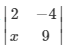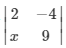mycollegehive
If  = 58 , find the value of x
utme-mathematics-2019 jamb jamb-2019 jamb-mathematics-2019 math matrix0If= 58 , find the value of x

262 viewsShareFollowSchool not set Nigeria
25 August 2020University of Lagos Nigeria
26 August 20200The above is a 2x2 matrix and the determinant is given as:

(2 * 9) - (-4 * x) = 58

18 - (-4x) = 58

18 + 4x = 58

4x = 58 - 18

4x = 40

x = 40/4

x = 10Share

### Groups

How to insert math formulas/equations### Related Tags

utme-mathematics-2019

5 followers

16 questionsjamb

7 followers

16 questionsjamb-2019

3 followers

16 questionsjamb-mathematics-2019

4 followers

16 questionsmath

43 followers

1302 questionsmatrix

3 followers

1 questions